## Use Cramers Rule to solve the system 2x – 3y = 11 -6x + 8y = 34

Question

Use Cramers Rule to solve the system
2x – 3y = 11
-6x + 8y = 34

in progress 0
7 months 2021-08-11T00:07:22+00:00 2 Answers 3 views 0

(x , y ) = ( -95, -67)

Step-by-step explanation:

Given system :-

2x – 3y = 11

-6x + 8y = 34

Find :- Solutions of system by using Cramers rule.

Solution :-

To solve the system using Cramer’s rule, list all needed determinants.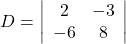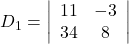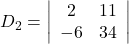• Evaluate the determinants.

D = 8 × 2 – ( -3) ( -6 )

= 16 – 18 = -2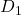= 11 × 8 – ( -3 ) ( 34 )

= 88 + 102 = 190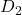= 2 × 34 – ( 11 ) ( -6 )

= 68 + 66 = 134

• Since D ≠ 0, Cramer’rs rule can be applied , so find x , y using the formulas;- x =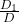, y =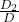.

Plug the value into the formula:-

x =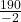, y =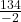.

Divide

x = -95 , y = -67

• The possible solution of the system is the ordered pair ( x , y ).

(x , y ) = ( -95, -67)

2. Step-by-step explanation:

given :

2x – 3y = 11

-6x + 8y = 34

find : the solutions of the system by using Cramers Rule.

solutions:

in the matrix 2×2 form =>

[ 2 -3] [x] 

=

[-6 8] [ y] 

D =

| 2 -3 |

|-6 8 |

= 8×2 – (-3) (-6)

= 16-18 = -2

Dx = | 11 -3 |

| 34 8 |

= 11×8 – (-3) (34)

= 88 + 102

= 190

Dy = | 2 11 |

|-6 34 |

= 2×34 – (-6) (11)

= 68 + 66

= 134

x = Dx/D = 190/-2 = 95

y = Dy/D = 134/-2 = 67

the solutions = {-95, 67}